# Introduction to ChIPSChIPS Threads (CIAO 4.11 ChIPS v1)

## Overview

#### Synopsis:

ChIPS is designed so that visualizations can be built up interactively and can easily be saved, printed, and restored. Visualizations may be created from FITS or ASCII format files, and include support for curves, histograms, contours and images.

Last Update: 15 Dec 2015 - Updated for CIAO 4.8

## Getting Started

The Starting ChIPS thread describes how to start ChIPS.

The data used in this thread is available in the chips_data.tar.gz file.

```unix% ls -1 chips/intro/
aa4059_chandra.fits
a4059_nvss.fits
curve_1.fits
curve_2.fits
example.state
hist.dat
hist_b.dat
lc.fits
spectrum.fits
```

In CIAO 4.8 the only WCS projection that is supported is the tangent-plane projection. This has meant that the SIN projection used for the NVSS observation of A4059 has been remapped - using the remap tool from the WCSTools package - to create the a4059_nvss.remap.fits file.

## Setting Preferences

After starting the ChIPS session, the following preferences were set to control the output size of the plots and hardcopies (this is not needed to create a visualization; it only needs to be done if you want to create the same size plots as used in this thread).

```chips> set_preferences(["window.width", 500, "window.height", 500])
chips> set_preferences(["export.pagewidth", 500, "export.pageheight", 500, "export.pageunits", "pixels"])
```

The Printing section of this thread explains how the figures in this thread were created.

## Basic Plotting

The make_figure command creates a curve, image, contour, or histogram from the data provided. It is a convenient wrapper for the add_curve, add_histogram, add_image, add_contour and supporting commands. Axis labels and units are drawn if the information is available in the input file. Plot titles are set to the value of the file keyword OBJECT or TITLE, or from the input filename.

### Spectrum

In this example, a FITS file containing a spectrum is used as input to make_figure, which creates Figure 1:

```chips> make_figure("spectrum.fits[cols channel,counts]")
```

### Figure 1: Spectrum plot created by make_figure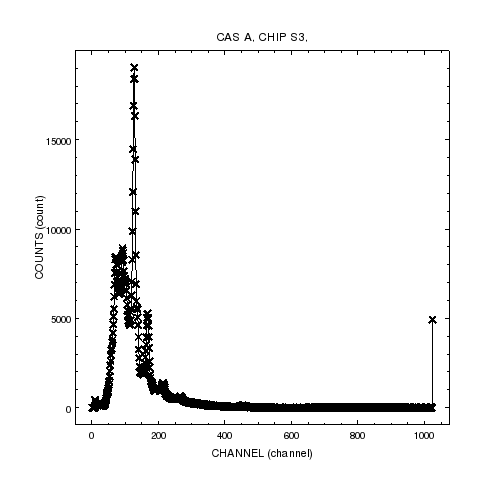### Figure 1: Spectrum plot created by make_figure

The channels and counts columns from spectrum.fits are plotted on the x and y axes respectively. The name and units of the axes were taken from the FITS file column information, and the plot title is set to the OBJECT header keyword value.

The same figure can be created using add_curve by saying:

```chips> clear()
chips> set_plot_title("CAS A, CHIP S3")
chips> set_plot_xlabel("CHANNEL (channel)")
chips> set_plot_ylabel("COUNTS (count)")
```

The plot can be further customized with the set_curve command. The following change the range of the x-axis, the line color, and the symbol size. The result is shown in Figure 2.

```chips> limits(X_AXIS, 0, 250)
chips> set_curve(["line.color", "olive", "symbol.size", 2])
```

### Figure 2: Modified spectrum plot### Figure 2: Modified spectrum plot

The plot contents have been changed from Figure 1: the line connecting the points of the curve is now drawn in olive, the symbol sizes are smaller, and the X-axis has been zoomed in to the range 0 to 250.

The ChIPS GUI makes it easy to modify the attributes of, and add annotations such as labels and lines to a, visualization. As described in the ChIPS GUI section of the 'Starting ChIPS' thread, the GUI can be displayed by

1. entering

`chips> show_gui()`

at the prompt,

2. or using the right-mouse-button menu in the ChIPS window and then selecting the Show GUI option.

The GUI can be closed down and re-started during a ChIPS session, or left running whilst you delete, create, and edit plots. For the plot shown in Figure 2, the ChIPS GUI looks like Figure 3.

### Figure 3: ChIPS GUI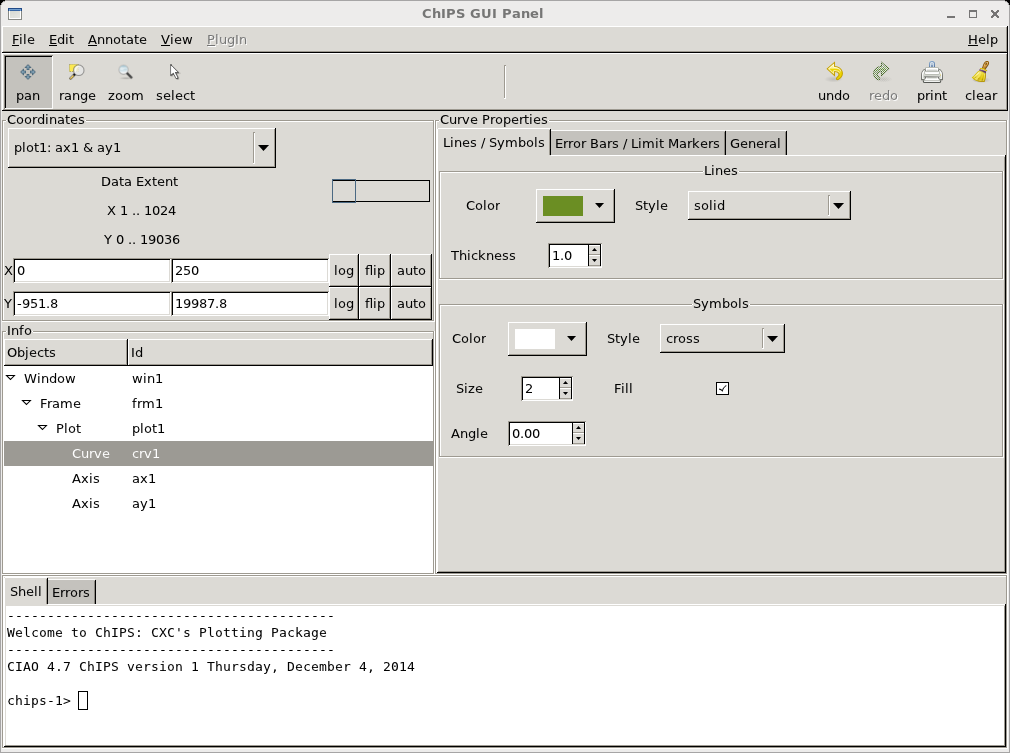### Figure 3: ChIPS GUI

The curve has been selected in the GUI so that you can easily edit its attributes, such as line color or symbol style. Changes in the GUI are immediately made to the visualization, and any changes made from ChIPS are automatically updated in the GUI.

### Lightcurve

A similar command can be used with a lightcurve file. Before plotting the lightcurve, clear the ChIPS window (or use add_window to create a new window), shown in Figure 4.

```chips> clear()
chips> make_figure("lc.fits[cols time,count_rate,count_rate_err]", "line.color=red err.style=cap err.color=plum")
```

### Figure 4: A lightcurve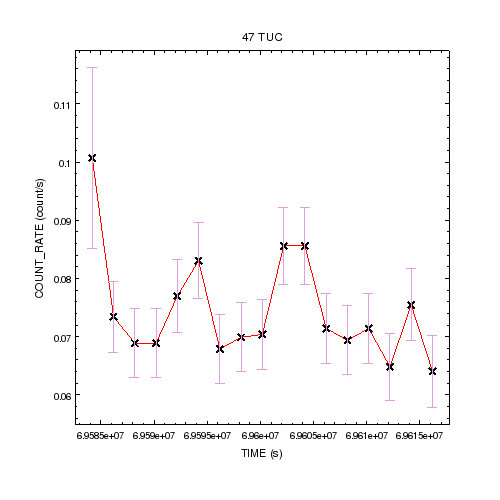### Figure 4: A lightcurve

The third column given to make_figure (count_rate_err) is used as symmetrical Y errors; see ahelp add_curve for more information on plotting errors. Also, several attributes are given in an attribute string, rather than as a list of values as used with the set_curve command above.

The same figure can be created using add_curve with the following:

```chips> add_window()
chips> add_curve("lc.fits[cols time,count_rate,count_rate_err]", "line.color=red err.style=cap err.color=plum")
chips> set_plot_title("47 TUC")
chips> set_plot_xlabel("TIME (s)")
chips> set_plot_ylabel(r"COUNT\_RATE (count/s)")
```

The underscore character in the y-axis label has been preceeded by a \ character to avoid it being interpreted as the LaTeX start subscript marker; this means that the string is preceeded by a r character to avoid it being interpreted as introducing a control character (see the Python documentation for String Literals for more information, noting that we use Python 2, not Python 3, in CIAO 4.8).

You can toggle the error-bar display - error up, error down, or both. The following command creates Figure 5:

```chips> set_curve(["err.*", False])
```

The above is a short-form, introduced in CIAO 4.2, which means the same:

```chips> set_curve(["err.down", False, "err.up", False])
```

### Figure 5: Removing the error bars### Figure 5: Removing the error bars

Both the upper and lower error bars from Figure 4 have been turned off. The Y-axis retains the same range as before because the automatic-limits calculation does not take into account whether the error bars are hidden or not.

### Histogram

ASCII data can also be used as input. The make_figure "figuretype" argument is set to "histogram" to force the figure to be drawn as a histogram.

```chips> !less hist.dat
40560        36.3
42438        34.7
44316        26.3
46194        22.9
48071        22.1
49949        13.1
50888        14.4
...
```
```chips> clear()
chips> make_figure("hist.dat","histogram")
```

The resulting plot is shown in Figure 6.

### Figure 6: A histogram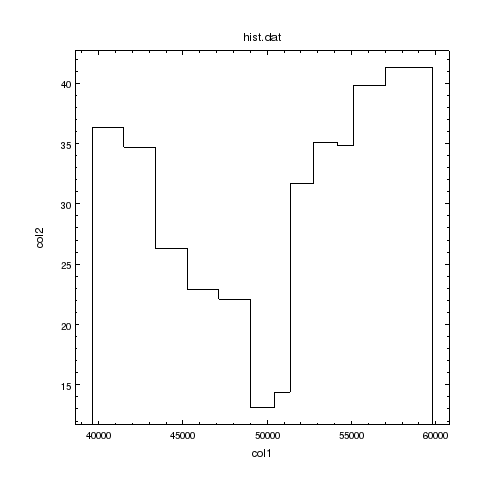### Figure 6: A histogram

The bins widths have been automatically calculated from the first column, which is assumed to give the center of each bin.

The same figure can be created using add_histogram with the following:

```chips> add_window()
chips> set_plot_title("hist.dat")
chips> set_plot_xlabel("col1")
chips> set_plot_ylabel("col2")
```

To fill the bins with the color "cornflower blue", say:

```chips> set_histogram(["fill.style", "solid", "fill.color","cornflower"])
chips> shuffle_histogram(chips_back)
```

which creates Figure 7. A range of fill patterns are available for histograms (and regions).

### Figure 7: A filled histogram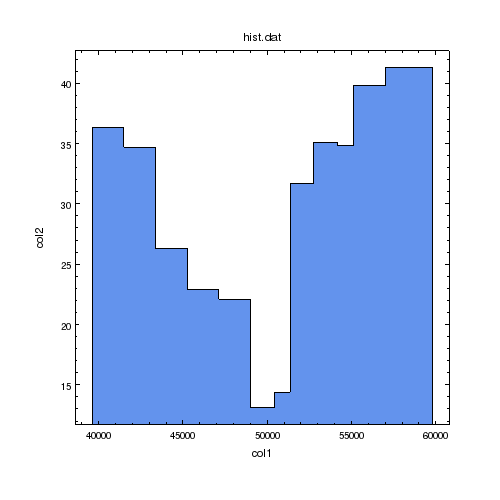### Figure 7: A filled histogram

The histogram is now filled by a light-blue color. The shuffle_histogram command is used to make sure that the tick marks on the X axis are not obscured by the histogram (it makes sure that the histogram is drawn before the X axis).

The following commands add a second histogram with vertical lines drawn at the edge of each bin. In this histogram the fill color is set to red and the opacity set to 0.5, as shown in Figure 8.

```chips> add_histogram("hist_b.dat", ["dropline", True, "fill.style", "solid", "fill.color", "red", "fill.opacity", 0.5])
chips> shuffle_axis("ax1", chips_front)
```

### Figure 8: Two filled histograms### Figure 8: Two filled histograms

The second histogram has been added to the plot. Since it also is drawn with a solid fill we change the order that the objects are drawn with the shuffle_axis command; rather than shift the order of the histograms, we move the X axis so that it is drawn last, so preserving the relative order of the two histograms (i.e. we want to keep the red histogram drawn on top of the blue one).

The patterns used for the histogram can be changed; here we change the solid fill to use diagonal lines for the two histograms, resulting in Figure 9.

```chips> set_histogram("hist1", ["fill.style", "updiagonal"])
chips> set_histogram("hist2", ["fill.style", "downdiagonal"])
```

### Figure 9: Two filled histograms (this time with lines)### Figure 9: Two filled histograms (this time with lines)

The histograms have been filled with lines; the first histogram (blue) uses diagonal lines going from bottom left to top right (the updiagonal pattern) whereas the second histogram (red) is filled with lines from top left to bottom right (the downdiagonal pattern).

In CIAO 4.8 the PNG and JPEG outputs may swap the updiagonal and downdiagonal patterns (i.e. the PNG version of this plot uses the downdiagonal pattern for the first/blue histogram).

### Image

In the following section we highlight some of the support for displaying image data in ChIPS.

```chips> clear()
chips> make_figure("a4059_chandra.fits", "image")
chips> set_image(["depth", 50])
chips> set_plot(["rightmargin", 0.15, "topmargin", 0.15])
```

The resulting plot is shown in Figure 10.

The images are - by default - created with an axis pad value of 0 (other objects, such as curves, histograms and contours still use the axis.pad value). This means that the image may hide part of the axes, unless you change the limits or change the relative ordering of the image and axes, as we do above by lowering the depth of the image.

### Figure 10: An image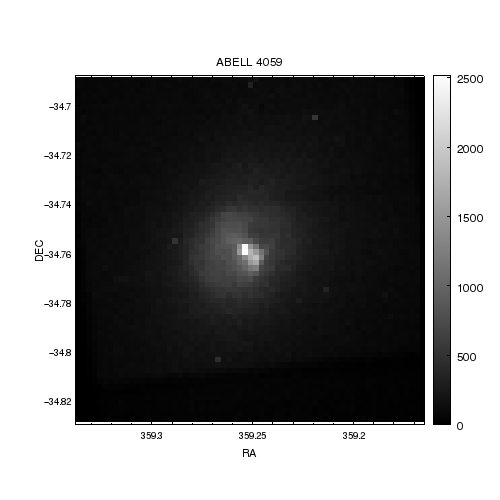### Figure 10: An image

The image is displayed using a linear scale, chosen to cover the full data range of the image. A colorbar has been added to the right-hand side of the plot to show the scaling of the pixel intensity values.

The right-hand margin of the plot is increased from the default value of 0.1 to 0.15 so that the colorbar labels do not fall off the edge of the display. Since the image is displayed with a fixed aspect ratio, we also change the top margin by the same amount so that the plot remains square.

The plot limits can be changed either from the ChIPS GUI or by interacting with the ChIPS window directly. For instance, the image can be scrolled around the plot by changing to the Pan mode and dragging around the image; alternatively the plot can be zoomed into using either the scroll wheel on your mouse (if one exists) or the + and - keys on your keyboard.

The same figure can be created using add_image with the following (note that we use the read_file command to read in the image since we will be accessing the pixel values below):

```chips> clear()
chips> set_plot(["rightmargin", 0.15, "topmargin", 0.15])
chips> set_plot_title("ABELL 4059")
chips> set_plot_xlabel("RA")
chips> set_plot_ylabel("DEC")
```

A number of colormaps are available, and can be selected by saying:

```chips> set_image(["colormap", "heat"])
```

and the axes can be changed to display sexagesimal notation with

```chips> set_xaxis(["tickformat", "ra"])
chips> set_yaxis(["tickformat", "dec"])
```

which creates Figure 11.

### Figure 11: Changing the colormap of an image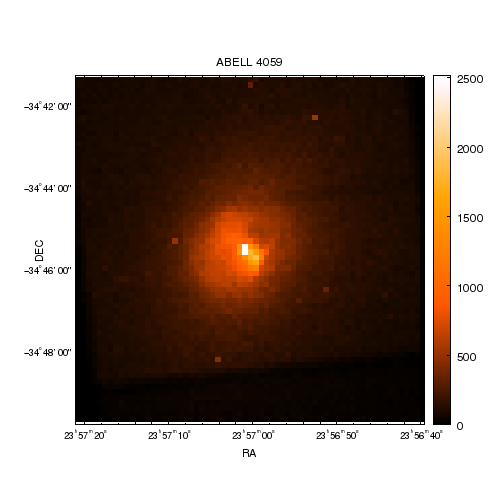### Figure 11: Changing the colormap of an image

The "heat" colormap is used to display the image; the colorbar scale has automatically changed to use it (from the grayscale used in Figure 10). The axis labels have been changed to display in sexagesimal, rather than decimal, notation.

We can change several attributes that control how the image is displayed; first we change from the default nearest-neighbor interpolation scheme to the bi-linear system:

```chips> set_image(["interpolation", "bilinear"])
```

We also wish to change the display range of the image, so we extract the pixel image values from the crate object cr and then use several NumPy routines to check the distribution of the pixel values.

```chips> ivals = copy_piximgvals(cr)
chips> ivals.min()
0
chips> ivals.max()
2517
chips> ivals.mean()
162.261538462
chips> np.median(ivals)
117.0
```

We can now change the threshold attribute of the image to change the display range; here we chose the values 100 to 2000.

```chips> set_image(["threshold", [100, 2000]])
```

The result of these changes is shown in Figure 12.

### Figure 12: Changing the interpolation and display range### Figure 12: Changing the interpolation and display range

The display has been changed to use the "bilinear" interpolation scheme (the default is "neighbor", a nearest-neighbor scheme) and to restrict the display range to 100 to 2000.

and the ChIPS gallery.

## Customizing the Figure

ChIPS allows users to control the appearance and behavior of objects - such as windows, curves, lines, images, contours, and axes - via configurable attribute settings. For instance, labels have font, color, font size, font style, angle, and alignment attributes. The attribute value can be set when the object is created or modified afterwards with a "set" command or via the ChIPS GUI. Please see the ChIPS GUI section of the 'Starting ChIPS' thread for more information on using the GUI to change the attributes of an object (or, better yet, start the GUI and try it out for yourself).

A set command exists for every ChIPS object:

As an example, if you have a curve and wish to change it to be drawn with red circles, connected by a green dottted line, you can say:

```chips> set_curve(["symbol.color", "red", "symbol.style", "circle", "line.color", "green", "line.style", "dot"])
```

The undo() and redo() routines allow you to easily recover from accidental or unwanted changes when changing attributes.

There are a few values that cannot be accessed via a set_<object> command. The most frequently-used of these are:

• set_plot_title: add a title to the plot
• set_plot_xlabel: label the x-axis
• set_plot_ylabel: label the y-axis

The characteristics of the labels and title may be modified by set_plot and set_axis:

```chips> set_plot(["title.font", "courier", "title.fontstyle", "bold"])
chips> set_axis(["label.fontstyle", "italic", "label.size", 20])
```

The set_axis command operates on both axes by default. An axis id can be specified to modify the x or y-axis, or use the set_xaxis and set_yaxis commands instead.

The ChIPS concept ahelp documents have more information on how to set attributes and allowed attribute values.

### Figure 13: The axis editor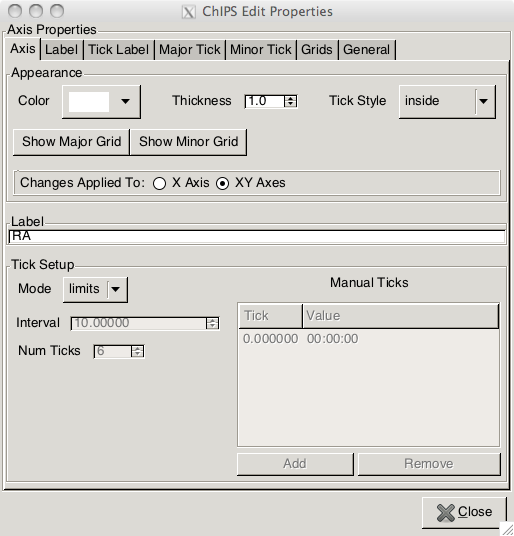### Figure 13: The axis editor

The Edit <Object> entry in the RMB menu will create - if the main GUI is not displayed - a window which allows you to edit the properties of the object. In this case the X axis of Figure 10 was selected. This is also available from the main GUI in the "Properties Frame".

The main attributes are presented on the first tab. For the case of axes you can also chose to change the partner axis at the same time.

## Overplotting Two Datasets

One limiting feature of make_figure is that it clears the current plot area before creating the figure; this means the make_figure cannot be used to add extra curves or histograms to an existing plot. It can be used to create the first curve, but then add_curve (or another add command) much be used to include additional curves.

To display two datasets in the same drawing area, simply use two add_curve commands. An attribute list is used to change the color and style of the lines, to tell them apart easily.

```chips> clear()
```

When the second curve is added, the y-axis is adjusted automatically to accomodate the full range of data, as shown in Figure 14.

### Figure 14: Two curves in the same plot### Figure 14: Two curves in the same plot

The second curve - drawn using the purple, dashed, line - has been added to the same plot as the first curve.

We now add some descriptive text to the plot: first we annotate the plot axes and title:

```chips> set_plot_title("Lightcurves of a Variable Source")
chips> set_plot_xlabel("Time (s)")
chips> set_plot_ylabel("Count Rate (s^{-1})")
```

and then we add labels to the plot itself with:

```chips> add_label(0.1, 0.4, "Curve 1", "color=red size=16 coordsys=PLOT_NORM")
chips> add_label(0.1, 0.3, "Curve 2", "color=purple size=16 coordsys=PLOT_NORM")
```

where the labels are positioned using the plot-normalized coordinate system, which has (0,0) at the bottom-left of the plot area and (1,1) at the top-right of the plot area.

We finish off by changing the angle and alignment of the tick labels on the X-axis. The axis-label offset is also adjusted so that the numeric tick labels do not overlap the axis label. Since this moves the axis label close to the bottom of the window, we increase the size of the bottom margin of the plot. The result is shown in Figure 15.

```chips> set_xaxis(["ticklabel.angle", 315, "ticklabel.halign", "left"])
chips> set_xaxis(["tickformat", "%.4z", "offset.perpendicular", 75])
chips> set_plot(["bottommargin", 0.2])
```

### Figure 15: Customising the plot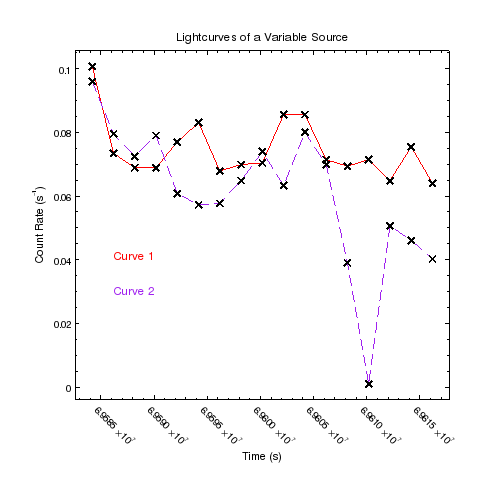### Figure 15: Customising the plot

The X-axis has been adjusted so that the tickmark labels are visible by rotating them, moving the axis label further from the axis, and re-sizing the plot by increasing the size of the bottom margin.

Data - such as contours, curves, and histograms - can be added on top of images. Figure 16 shows the NVSS data of Abell 4059 overlain onto the Chandra image, and was created with the following commands:

```chips> clear()
chips> make_figure("a4059_chandra.fits", "image", ["colormap", "hsv"])
chips> set_image(["depth", 50])
chips> add_contour("a4059_nvss.remap.fits", [0.05, 0.1, 0.3, 0.5], ["color", "red", "thickness", 2])
```

In CIAO 4.8, the only supported WCS projection is the tangent-plane system. Attempts to use an un-supported projection will result in the following warning message being displayed:

```chips WARNING: Unable to use world transform - RA---SIN, DEC--SIN is an unsupported type. Using physical transform.
```

As discussed above the NVSS data has been re-mapped onto the tangent-plane projection to allow it to be used here in the add_contour routine.

```chips> set_axis(["majorgrid.visible", True, "majorgrid.color", "orange"])
chips> panto(359.2539, -34.76148)
chips> zoom(1.5)
chips> set_yaxis(["offset.perpendicular", 50])
```

In this visualization we have also added in display of the axis grid lines - by setting majorgrid.visible to True - and changed the area being displayed; the panto call changes where the display is centered and zoom the axis limits. The change to the offset.perpendicular attribute of the Y axis moves the label (DEC) away from the numeric labels of the axis.

As discussed when creating Figure 10, the ChIPS GUI - in particular the direct interaction with the ChIPS window provides an alternative method to the command line for changing the display area of a plot.

### Figure 16: Combining contour and image plots### Figure 16: Combining contour and image plots

The radio emission is shown in red contours, on top of the Chandra image. Since the contours were added after the axes, they are drawn above the orange grid lines; the image is drawn below these grid lines since its depth (50) is lower than the axes, which has the default value of 100.

## Plotting Two Datasets Side-by-Side

It is also possible to create multiple plotting areas within a ChIPS window, if you want the curves to be side-by-side instead of overlaid. This is done with the split command.

Note that split is only one method of creating multiple plotting areas in ChIPS. Another is the strip_chart command, which can create an arrangement of plotting areas with different sizes and synchronized axes. It is also possible to create and arrange multiple plotting areas with the add_plot and move_plot commands, but using split or split_chart is much simpler. Further examples of these techniques are available in the creating and using multiple plots thread.

If no argument is given, a 2 x 1 grid is created with no gap between the plotting areas. For this example, we create a 2 x 1 grid with a small gap:

```chips> clear()
chips> split(2, 1, 0.05)
```

### Figure 17: Two empty plot areas### Figure 17: Two empty plot areas

There are now two plot areas (or "plots") that can be used for displaying curves, histograms, or contours. The output of the info command, shown below, shows that the top plot - which has coordinates of (0.15,0.55) to (0.90,0.90) - is called plot1, whilst the bottom plot is called plot2. The coordinate system used for plot positions has (0,0) at the bottom-left and (1,1) at the top right of the frame.

There are now two plots, as shown in Figure 17; the plot named "plot1" is current:

```chips> print(info())
Window [win1]
Frame [frm1]
Plot [plot1]   (0.15,0.55)  .. (0.90,0.90)
Border bottom [bx1]  top [bx2]  left [by1]  right [by2]
Plot [plot2]   (0.15,0.15)  .. (0.90,0.50)
Border bottom [bx1]  top [bx2]  left [by1]  right [by2]

chips> print(info_current())
Window [win1]
Frame [frm1]
Plot [plot1]
Coord Sys [Plot Normalized]

```

In the commands below, the first curve is put in the current plot (plot1, the upper plot). It is then necessary to specify where the second curve should go, which we do by using the current_plot command:

```chips> add_curve("curve_1.fits[cols time,count_rate]")
chips> current_plot("plot2")
```

The result is shown in Figure 18.

### Figure 18: Adding curves to the plots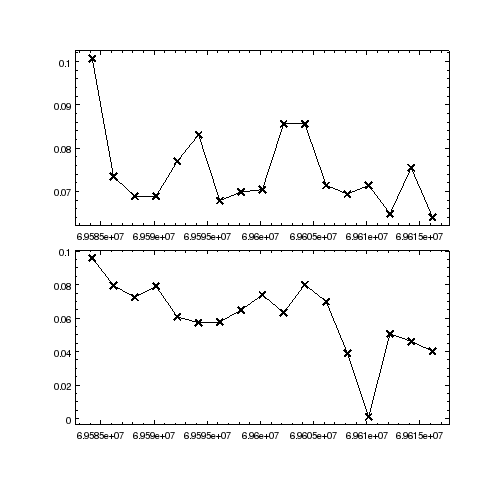### Figure 18: Adding curves to the plots

The top plot contains the data from the file curve_1.fits and the bottom plot the file curve_2.fits.

Various modifications are now made to the plots. Note that multiple objects can be modified at once by setting the currency to "all" (for example, the Y label of both plots can be changed with a single call).

```chips> current_plot("all")
chips> set_curve(["line.color", "red", "symbol.style", "circle"])
chips> set_plot_ylabel("Count Rate (cts/s)")

chips> set_plot_title("plot1","Lightcurves of a Variable Source")

chips> set_plot_xlabel("plot2", "Time (s)")
```

The result is shown in Figure 19.

### Figure 19: Annotating the plots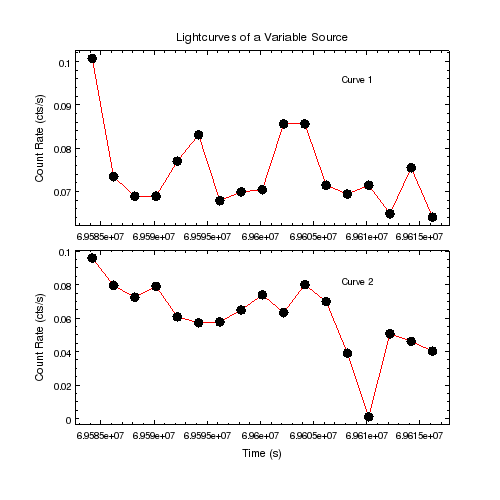### Figure 19: Annotating the plots

Customisations can be made to each plot individually, such as adding the labels Curve 1 and Curve 2, or to both at the same time, such as the Y-axis label or changing the attributes of the curves.

and the ChIPS gallery.

## Printing

The print_window command prints the contents of the window to a file (if a filename is specified) or to the printer. Available formats are PS (the default setting), EPS, PDF, PNG, and JPG.

### Send straight to the printer

To print the current window to the default printer use the print_window call with no arguments (this assumes that the export.printdialog preference setting is false, which is the default value):

```chips> print_window()
```

If the export.printdialog preference setting is set to true then this call will create a GUI which can be used to control the output.

### Save as a file (PS, EPS, PDF, PNG, JPEG formats)

To save the current window as a postscript file, PNG file, and a JPG image (fig1.ps, fig1.png and fig1.jpg respectively):

```chips> print_window("fig1")
chips> print_window("fig1.png")
chips> print_window("fig1", ["format","jpg"])
```

The first print_window command uses the default format value. This is "ps", unless the user has changed it with a set_preference command.

As when sending the plot straight to the printer, a GUI will be created if the printdialog preference is set to true.

### Using a GUI to control the print output

If the export.printdialog preference setting is set to true (the default is false) then calling print_window will create a GUI which can be used to select the plot options. So

```chips> print_window("fig1", ["printdialog", True])
```

will create Figure 20.

This dialog window can also be called up directly from the ChIPS window, via the right-mouse-button menu, or from the Print options in the ChIPS GUI, as discussed in the Starting ChIPS thread.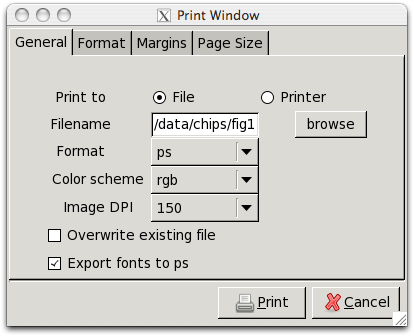### Figure 20: GUI for print_window

The GUI can be used to change the print options - such as output format, page size (for ps and PDF formats), margins, location of the output file, and more.

### How are on-screen colors changed on print out?

The default color value for most items is set to default, which means that it will appear in white on screen, but be in black in hardcopy form (i.e. any format created by the print_window form). In this thread, the figures show the screen output (and so have a black background with white lines), and the postscript versions of the plot have the reverse of this (black lines on a white background). The "PNG" link in Figure 19 shows the PNG output created by the call

```chips> print_window("multiplot-final.png)
```

and so has a white background with lines drawn in black (other than the curve line, which was explicitly changed to red).

### What else can be changed?

There are a number of attributes to the print_window command which control the appearance of the output plot: page size, margins, color system, etc. Refer to the help file for a list.

These options can also be explored by using the GUI when calling print_window.

## Saving and Restoring the Session

There are three ways to save the current ChIPS visualization:

### Using the make_script() command

The make_script command creates a Python script which can be used to re-create the current set of ChIPS plots. The commands are not guaranteed to be the same as those used to create the plots, unlike the binary format.

```chips> make_script("intro.py")
```

The script can be read, and edited; the start of the file for this particular case looks like:

```from pycrates import *     # import crates i/o routines
from pychips.all import *  # import chips hlui and advanced commands

### Data Templates
# The template variables below are used to specify the data needed for
# objects such as curves. The values may be substituted with other file
# names or data arrays as appropriate. It is the user's responsibility to
# ensure that data arrays are all the correct length, that provided column
# names exist, and that if files are provided, they contain the appropriate
# columns in the correct order. Note that the variables are provided in two
# flavors- as filenames and as data structures: the former makes use of the
# simpler file interface while the latter offers more flexibility.
class DataObj(): pass

MyCrate_1 = TABLECrate("intro.py.Data1_curve.fits")
MyData_1 = DataObj()  # data- intro.py.Data1_curve.fits
MyData_1.xx    = MyCrate_1.get_column("xx").get_values()
MyData_1.yy    = MyCrate_1.get_column("yy").get_values()

MyCrate_2 = TABLECrate("intro.py.Data2_curve.fits")
MyData_2 = DataObj()  # data- intro.py.Data2_curve.fits
MyData_2.xx    = MyCrate_2.get_column("xx").get_values()
MyData_2.yy    = MyCrate_2.get_column("yy").get_values()

open_undo_block()          # treat script as single command
clear()                    # remove all existing chips objects

# This script assumes that the user's default preferences were used
# to generate the output and were not changed- so the following
# load_preferences() command is unnecessary. To guarantee that this
# script uses the correct settings to replicate the saved state,
# uncomment out the following line.

add_plot(0.15,0.55,0.90,0.90," title.halign=0.5 title.valign=0.5 title.label=\"Lightcurves of a Variable Source\"")
...
```

This file can be used when starting ChIPS:

```unix% chips intro.py
```

or from within a ChIPS session:

```chips> execfile("intro.py")
```

### Using the save_state() routine

The save_state command saves the current ChIPS session into a binary format, platform-independent, state file.

```chips> save_state("intro.state")
```

The saved session can be restored with the load_state command:

```chips> load_state("intro.state")
```

The state file is in binary format - and so is not human readable - and includes the data needed to re-create the visualization, as well as a record of the history buffer, so you can use undo() and redo() even if the state file has been loaded into a new ChIPS session, even on a different machine.

Note that load_state will delete any existing plots in the current ChIPS session and that the pre-existing visualization can not be restored using undo.

State files from recent CIAO releases can be loaded into CIAO 4.8, but files created in CIAO 4.8 may not be read back into earlier versions. If this is the case then the following error message will be raised:

```chips> load_state("example.state")
chips ERROR: Unable to load incompatible state file (unsupported major version)
```

### Using the File/Save As menu item of the GUI

The ChIPS GUI allows you to create either a Python file (output of make_script) or a binary state file (output of save_state) by using the File/Save As menu item.

## History

 22 Aug 2007 new for CIAO 4.0 [Beta 2] 10 Jul 2008 added example.state file to tarfile; added links to new threads; figures are now included within the thread 15 Dec 2008 CIAO 4.1: fill patterns for histograms (e.g. Figure 9); when printing you can now use a GUI to select the options; updated the state file as it is not backwards-compatible with CIAO 4.0 15 Dec 2009 Updated for CIAO 4.2; added examples of the new image support, contour use, and a discussion of the new make_script() command. 15 Dec 2010 Updated for CIAO 4.3: the NVSS image has been converted to a tangent-plane projection since the SIN projection is not supported in CIAO 4.3; images are now created with an axis.pad value of 0; added an extra visualization (Figure 12) showing how the image interpolation and threshold attributes can be changed; axes grid lines have been added to the combined image and contour (Figure 16) figure, as well as examples of the new zoom and panto commands. 15 Dec 2011 Updated for CIAO 4.4: noted the improved PDF support (e.g. Figure 8 for displaying opaque/transparent region, histograms, and images, Figure 10 for the improved color-bar display); highlighted the new ChIPS GUI in multiple places, including the Using the print GUI section and the new File/Save As section. 13 Dec 2012 Updated for CIAO 4.5 04 Dec 2013 Updated for CIAO 4.6 09 Dec 2014 Updated for CIAO 4.7 18 Mar 2015 Updated lightcurve file name to match what is provided in the data files tarball. 15 Dec 2015 Updated for CIAO 4.8